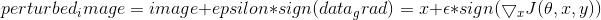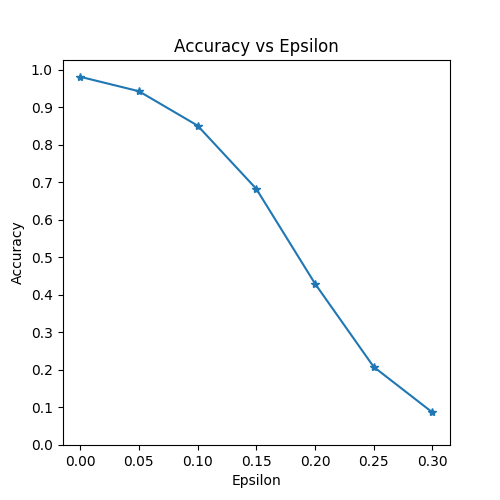生成对抗示例

1.威胁模型

FGSM 攻击是一种白盒攻击，其目标是错误分类。有了这些背景信息，我们现在可以详细讨论攻击。3.实现

3.1 引入相关包

from __future__ import print_function
import torch
import torch.nn as nn
import torch.nn.functional as F
import torch.optim as optim
from torchvision import datasets, transforms
import numpy as np
import matplotlib.pyplot as plt

3.2 输入

epsilons = [0, .05, .1, .15, .2, .25, .3]
pretrained_model = "data/lenet_mnist_model.pth"
use_cuda=True

3.2 被攻击的模型

# 定义LeNet模型
class Net(nn.Module):
def __init__(self):
super(Net, self).__init__()
self.conv1 = nn.Conv2d(1, 10, kernel_size=5)
self.conv2 = nn.Conv2d(10, 20, kernel_size=5)
self.conv2_drop = nn.Dropout2d()
self.fc1 = nn.Linear(320, 50)
self.fc2 = nn.Linear(50, 10)

def forward(self, x):
x = F.relu(F.max_pool2d(self.conv1(x), 2))
x = F.relu(F.max_pool2d(self.conv2_drop(self.conv2(x)), 2))
x = x.view(-1, 320)
x = F.relu(self.fc1(x))
x = F.dropout(x, training=self.training)
x = self.fc2(x)
return F.log_softmax(x, dim=1)

#声明 MNIST 测试数据集何数据加载
transforms.ToTensor(),
])),
batch_size=1, shuffle=True)

# 定义我们正在使用的设备
print("CUDA Available: ",torch.cuda.is_available())
device = torch.device("cuda" if (use_cuda and torch.cuda.is_available()) else "cpu")

# 初始化网络
model = Net().to(device)

# 加载已经预训练的模型

# 在评估模式下设置模型。在这种情况下，这适用于Dropout图层
model.eval()
• 输出结果：
Extracting ../data/MNIST/raw/train-images-idx3-ubyte.gz
Extracting ../data/MNIST/raw/train-labels-idx1-ubyte.gz
Extracting ../data/MNIST/raw/t10k-images-idx3-ubyte.gz
Extracting ../data/MNIST/raw/t10k-labels-idx1-ubyte.gz
Processing...
Done!
CUDA Available:  True

3.3 FGSM算法攻击# FGSM算法攻击代码
# 收集数据梯度的元素符号
# 通过调整输入图像的每个像素来创建扰动图像
# 添加剪切以维持[0,1]范围
perturbed_image = torch.clamp(perturbed_image, 0, 1)
# 返回被扰动的图像
return perturbed_image

3.4 测试函数

def test( model, device, test_loader, epsilon ):

# 精度计数器
correct = 0

# 循环遍历测试集中的所有示例

# 把数据和标签发送到设备
data, target = data.to(device), target.to(device)

# 通过模型前向传递数据
output = model(data)
init_pred = output.max(1, keepdim=True) # get the index of the max log-probability

# 如果初始预测是错误的，不打断攻击，继续
if init_pred.item() != target.item():
continue

# 计算损失
loss = F.nll_loss(output, target)

# 将所有现有的渐变归零

# 计算后向传递模型的梯度
loss.backward()

# 唤醒FGSM进行攻击

# 重新分类受扰乱的图像
output = model(perturbed_data)

# 检查是否成功
final_pred = output.max(1, keepdim=True) # get the index of the max log-probability
if final_pred.item() == target.item():
correct += 1
# 保存0 epsilon示例的特例
if (epsilon == 0) and (len(adv_examples) < 5):
else:
# 稍后保存一些用于可视化的示例

# 计算这个epsilon的最终准确度
print("Epsilon: {}\tTest Accuracy = {} / {} = {}".format(epsilon, correct, len(test_loader), final_acc))

# 返回准确性和对抗性示例

3.5 运行攻击

accuracies = []
examples = []

# 对每个epsilon运行测试
for eps in epsilons:
acc, ex = test(model, device, test_loader, eps)
accuracies.append(acc)
examples.append(ex)
• 输出结果：
Epsilon: 0      Test Accuracy = 9810 / 10000 = 0.981
Epsilon: 0.05   Test Accuracy = 9426 / 10000 = 0.9426
Epsilon: 0.1    Test Accuracy = 8510 / 10000 = 0.851
Epsilon: 0.15   Test Accuracy = 6826 / 10000 = 0.6826
Epsilon: 0.2    Test Accuracy = 4301 / 10000 = 0.4301
Epsilon: 0.25   Test Accuracy = 2082 / 10000 = 0.2082
Epsilon: 0.3    Test Accuracy = 869 / 10000 = 0.0869

4.结果

4.1 准确度 vs Epsilon

plt.figure(figsize=(5,5))
plt.plot(epsilons, accuracies, "*-")
plt.yticks(np.arange(0, 1.1, step=0.1))
plt.xticks(np.arange(0, .35, step=0.05))
plt.title("Accuracy vs Epsilon")
plt.xlabel("Epsilon")
plt.ylabel("Accuracy")
plt.show()4.2 样本对抗性示例

# 在每个epsilon上绘制几个对抗样本的例子
cnt = 0
plt.figure(figsize=(8,10))
for i in range(len(epsilons)):
for j in range(len(examples[i])):
cnt += 1
plt.subplot(len(epsilons),len(examples),cnt)
plt.xticks([], [])
plt.yticks([], [])
if j == 0:
plt.ylabel("Eps: {}".format(epsilons[i]), fontsize=14)